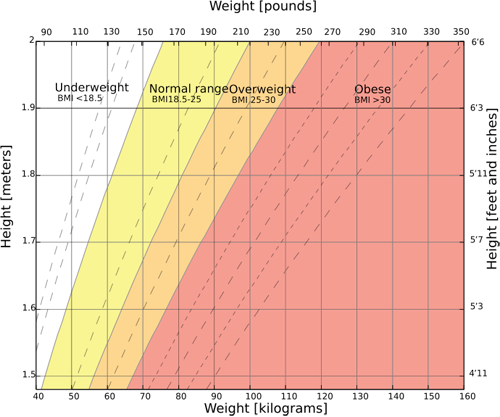# Body Mass Index Calculator

Please select the gender, enter the height and weight of the person in centimeters and in kilogram. Then click on the calculate button to calculate his/her body mass index.

Body Mass Index Calculation Form
1. Female Male
2. cm
3. kg

## What is body mass index?

BMI is a measure of someone's weight in relation to height.

## What is an ideal weight?

According to doctors an ideal weight would be inside the healthy weight range for women as defined by the Body Mass Index - i.e. a BMI between 19 and 24.9. (See Figure below)## How to calculate body mass index?

To calculate one's BMI, multiply one's weight in pounds and divide that by the square of one's height in inches.

## BMI Categories

• <18.5: Underweight
• 18.5-24.9: Normal weight
• 25-29.9: Overweight
• 30+: Obesity